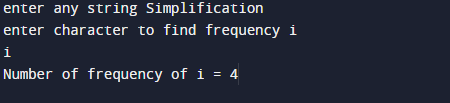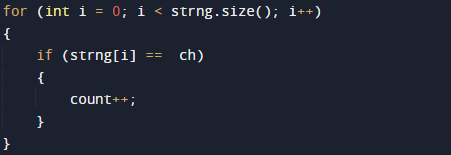# C++ Program to Find the Frequency of Characters in a String

In this tutorial you will learn about the C++ Program to Find the Frequency of Characters in a String and its application with practical example.

In this tutorial, we will create a c++ program to  find frequency of occurrence of a character in a String  using C++ programming language.

## Prerequisites

Before starting with this tutorial we assume that you are best aware of the following C++ programming topics:

• Operators.
• looping statements.
• Basic input/output.
• Basic c++ programming
• For Loop.
• String.

## What Is frequency of occurrence of a character ?

frequency means  to find and count  given  character present in the string.i.e total number of occurrence of a particular character in a string.

## C++ Program to Find the Frequency of Characters in a String.

In this program we will we will find frequency of a given character. We would first declared and initialized the required variables. Next, user will be prompted to enter a string and a character to find the frequency of given character.

## OutputIn the above program, we have first declared and initialized a set variables required in the program.

• strng = it will hold entered string.
• ch = it will hold a finding character.
• i=  for iteration.
• count= count number of occurrence of a character.

And  in the next statement user will be prompted to enter string and a character  which will be assigned to variable ‘strng’ and in ‘ch’ respectively. Now we will loop through the size of  a string  using sizeof() function in each  iteration we will compare string to given character to find the occurrence of a character and each matching condition we increase the counter value by  one as shown in  image.This process  will continues until the loop ends , and at last we will print the total number  of  occurrence of a given character in the string.This is the process  of finding  the frequency of occurrence of a character in a String.

In this tutorial we have learn about the C++ Program to Find the Frequency of Characters in a String and its application with practical example. I hope you will like this tutorial.